#### An integral curve

If a vector field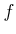is given, then a velocity vector is defined at each point using (8.10). Imagine a point that starts at some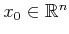at time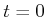and then moves according to the velocities expressed in. Where should it travel? Its trajectory starting from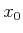can be expressed as a function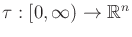, in which the domain is a time interval,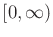. A trajectory represents an integral curve (or solution trajectory) of the differential equations with initial condition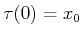if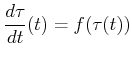(8.14)

for every time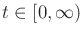. This is sometimes expressed in integral form as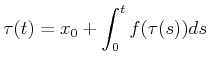(8.15)

and is called a solution to the differential equations in the sense of Caratheodory. Intuitively, the integral curve starts atand flows along the directions indicated by the velocity vectors. This can be considered as the continuous-space analog of following the arrows in the discrete case, as depicted in Figure 8.2b.

Example 8..9 (Integral Curve for a Constant Velocity Field)   The simplest case is a constant vector field. Suppose that a constant field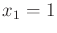and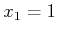is defined on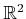. The integral curve from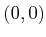is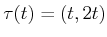. It can be easily seen that (8.14) holds for all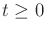.Example 8..10 (Integral Curve for a Linear Velocity Field)   Consider a velocity field on. Let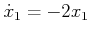and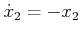. The function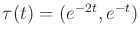represents the integral curve from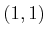. At,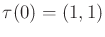, which is the initial state. If can be verified that for all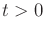, (8.14) holds. This is a simple example of a linear velocity field. In general, if each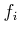is a linear function of the coordinate variables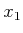,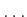,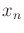, then a linear velocity field is obtained. The integral curve is generally found by determining the eigenvalues of the matrix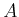when the velocity field is expressed as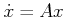. See  for numerous examples.A basic result from differential equations is that a unique integral curve exists to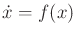ifis smooth. An alternative condition is that a unique solution exists ifsatisfies a Lipschitz condition. This means that there exists some constant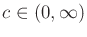such that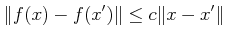(8.16)

for all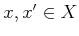, and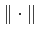denotes the Euclidean norm (vector magnitude). The constant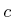is often called a Lipschitz constant. Note that ifsatisfies the Lipschitz condition, then it is continuous. Also, if all partial derivatives ofover all ofcan be bounded by a constant, thenis Lipschitz. The expression in (8.16) is preferred, however, because it is more general (it does not even imply thatis differentiable everywhere).

Steven M LaValle 2020-08-14